Short articles on topics in systematic trading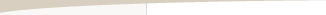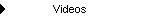Other Articles...

Monte Carlo analysisMonte Carlo Analysis

by Michael R. Bryant

Monte Carlo analysis is a computational technique that makes it possible to include the statistical properties of a model's parameters in a simulation. In Monte Carlo analysis, the random variables of a model are represented by statistical distributions, which are randomly sampled to produce the model's output. The output is therefore also a statistical distribution. Compared to simulation methods that don't include random sampling, the Monte Carlo method produces more meaningful results, which are more conservative and also tend to be more accurate when used as predictions.

When using use Monte Carlo analysis to simulate trading, the trade distribution, as represented by the list of trades, is sampled to generate a trade sequence. Each such sequence is analyzed, and the results are sorted to determine the probability of each result. In this way, a probability or confidence level is assigned to each result.

Without Monte Carlo analysis, the standard approach for calculating the historical rate of return, for example, would be to analyze the current sequence of trades using, say, fixed fractional position sizing. It might be found that the rate of return over the sequence was 114%. With Monte Carlo analysis, on the other hand, hundreds or thousands of different sequences of trades are analyzed, and the rate of return is expressed with a probability qualifier. For example, the rate of return as determined by Monte Carlo analysis might be 83% with 95% confidence. This means that of all the thousands of sequences considered, 95% had rates of return greater than or equal to 83%.

Monte Carlo analysis is particularly helpful in estimating the maximum peak-to-valley drawdown. To the extent that drawdown is a useful measure of risk, improving the calculation of the drawdown will make it possible to better evaluate a trading system or method. Although we can't predict how the market will differ tomorrow from what we've seen in the past, we do know it will be different. If we calculate the maximum drawdown based on the historical sequence of trades, we're basing our calculations on a sequence of trades we know won't be repeated exactly. Even if the distribution of trades (in the statistical sense) is the same in the future, the sequence of those trades is largely a matter of chance.

Calculating the drawdown based on one particular sequence is somewhat arbitrary. Moreover, the sequence of trades has a very large effect on the calculated drawdown. If you choose a sequence of trades where five losses occur in a row, you could get a very large drawdown. The same trades arranged in a different order, such that the losses are evenly dispersed, might have a negligible drawdown.

In using a Monte Carlo approach to calculate the drawdown, the historical sequence of trades is randomized, and the rate of return and drawdown are calculated for the randomized sequence. The process is then repeated several hundred or thousand times. Looking at the results in aggregate, we might find, for example, that in 95% of the sequences, the drawdown was less than 30% when 4% of the equity was risked on each trade. We would interpret this to mean that there's a 95% chance that the drawdown will be less than 30% when 4% is risked on each trade.

The benefit of selection without replacement is that it exactly duplicates the probability distribution of the input sequence, whereas selection with replacement may not. The drawback to selection without replacement is that the randomly sampled sequences are limited to the number of trades in the input sequence. If you have a short sequence of trades (say, less than 30 trades), this may limit the accuracy of certain calculations, such as the drawdown.

An example based on sampling without replacement is shown below. Trading is simulated using fixed ratio position sizing starting with an account equity of \$10,000. Each simulation employs 500 trade sequences (samples). The first results section in the figure shows key results, such as the rate of return, at a series of confidence levels. Notice, for example, that lower returns are predicted for higher confidence levels.Example of Monte Carlo analysis results.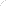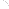Join Our Email List Email: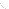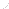For Email Marketing you can trust